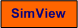# Level Control of Equalization Tank

Snapshot of the front panel of the simulator:## Description of the simulated system

The system being simulated is a level control system of a liquid tank. The controller function is PI (proportional plus integral), in the form of the LabVIEW PID Advanced function.

The mathematical model of the process to be controlled is based on mass balance of the contents, which is water, of the tank. The mass balance is as follows:

A*dh(t)/dt = Fin(t) - Fout(t)

The outflow Fout(t) is assumed to be the same as the pump control signal u(t) (both in unit of m3/s).

## Video

Here is an instructional video where a previous version of the present simulator is used as an example: Flow Smoothing with Buffer Tank.

## Aims

The aim of this simulator is to demonstrate the use of level control to obtain equalization (or buffering) of flow.

## Motivation

Flow equalization is important in e.g. wastewater treatment plants. Compliant or soft level control is used to obtain a proper equalization or smoothing of the varying inflow.